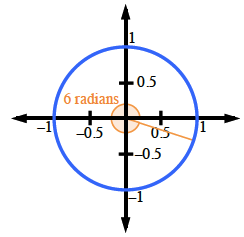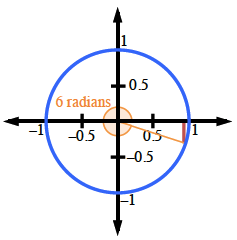### Home > INT3 > Chapter 9 > Lesson 9.1.6 > Problem9-80

9-80.

Sketch a unit circle with an angle that measures $6$ radians.

1. Approximately how many degrees is $6$ radians?

Notice that $2π$ radians $≈ 2\left(3.14\right) ≈ 6.27$.
So it's a little less than $360^\circ$.

$\frac{6}{2 \pi} = \frac{x}{360}$

$6 \text{ radians} ≈ 344^\circ$2. Using only your diagram, estimate the sine of $6$ radians.

sin$\left(6\right) = −0.3$